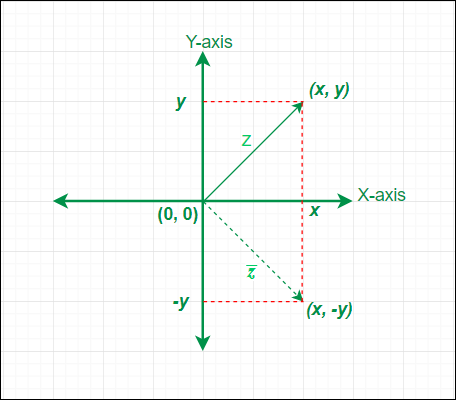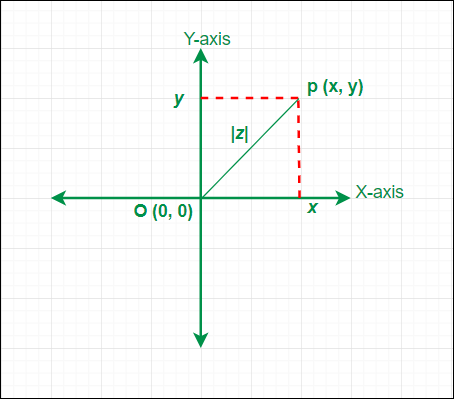# Complex Conjugate

• Difficulty Level : Easy
• Last Updated : 21 Feb, 2021

A complex number is a number represented in the form of (x + i y); where x & y are real numbers, and i = √(-1) is called iota (an imaginary unit). It is also known as imaginary numbers or quantities. A complex number can be purely real or purely imaginary depending upon the values of x & y.

Examples:

Attention reader! All those who say programming isn't for kids, just haven't met the right mentors yet. Join the  Demo Class for First Step to Coding Coursespecifically designed for students of class 8 to 12.

The students will get to learn more about the world of programming in these free classes which will definitely help them in making a wise career choice in the future.

(5 + 3 i), (0 + 7 i), (-4 + 9 i), (1 + 0 i), (0 – i), etc.

Now we will discuss two very important concepts of complex numbers which are Conjugate and Modulus.

## Conjugate of a Complex Number

Conjugate of a complex number is another complex number whose real parts Re(z) are equal and imaginary parts Im(z) are equal in magnitude but opposite in sign. Sometimes conjugate of a complex number is also called a complex conjugate. Conjugate of a complex number z is represented byand (z &) together known as complex-conjugate pair because z andare conjugate of each other. If z = (x + i y) is a complex number then conjugate of z is defined as= (x – i y). From the relation between z and, we can say conjugate of a complex is obtained by replacing i with (-i). The geometrical meaning of conjugate of a complex numberis the reflection or mirror image of the complex number z about the real axis (X-axis) in the complex plane or argand plane.Conjugate of a complex number in Argand Plane

### Properties of Conjugate

If z, z1, & z2 are complex numbers then they will have the following conjugate properties.

1. Conjugate of a purely real complex number is the number itself (z =) i.e. conjugate of (7 + 0 i) = (7 – 0 i) = 7
2. Conjugate of a purely imaginary complex number is negative of that number (z += 0) i.e. conjugate of (0 -7 i) = (0 + 7 i) = 7 i
3.= z
4. z += 2 Re(z)
5. z –= 2 i . Im(z)
6.= {Re(z)}2 + {Im(z)}2
7.=8.=9.=10. z = (z1 / z2) then=/; z2 ≠ 0

Note: In order to find out the conjugate of a complex number that complex number must be in its standard form that is Z = (x + i y). If the complex number is not in its standard form then it has to be converted into its standard form before finding its complex conjugate.

### Examples

Example 1: If z = (5 + 7 i) is a complex number then its conjugate is given by

Solution:= (5 – 7 i).

Example 2: If z = 1 / (4 + 3 i) is a complex number then its conjugate is given by

Solution:

First z has to be converted into its standard form by multiplying the numerator and

denominator with the conjugate of (4 + 3 i)

z = (1 / (4 + 3 i)) × ((4 – 3 i) / (4 – 3 i))

z = (4 – 3 i) / (16 + 9)

z = (4 / 25) – (3 / 25) i

The conjugate of z is= (4 / 25) + (3 / 25) i

Example 3: If (a + i b) is a complex number which is the complex conjugate of (8 – 3 i)  then values of a and b are  given by

Solution:

Let z = a + i b= (8 + 3 i)

z =z = (8 + 3 i)

Two complex numbers are equal only when their corresponding real & imaginary parts are equal

Equating the real and imaginary parts of z & (8 + 3 i)

Re(z) = a = 8

Im(z) = b = 3

Hence 8 & 3 are the respective values of a and b.

## Modulus of a Complex Number

The modulus of a complex number z = (x + i y) is defined as |z| = √(x2 + y2); where x = Re(z) and y = Im(z). |z| ≥ 0 for all complex numbers. We cannot compare two complex numbers z1 and z2 i.e. (z1 > z2) or (z1 < z2) has no significance but (|z1| > |z2|) or (|z1| < |z2|) has definite meaning because |z1| & |z2| are real numbers. The geometrical meaning of modulus of a complex number (x + i y) is the distance of the point P (x, y) from the origin O (0, 0) in the complex plane or argand plane i.e. |z| == √((x – 0)2 + (y – 0)2) = √(x2 + y2).Modulus of a complex number on Argand plane

### Properties of Modulus

If z, z1, z2 & zn are complex numbers then they will have the following modulus properties.

1. If a complex number z has equal real and imaginary parts then its modulus is zero i.e. Re(z) = Im(z) ⇒ |z| = 0
2. Real and imaginary part of a complex number z always lies between -|z| and  |z|  i.e. -|z| ≤ Re(z) ≤ |z| and -|z| ≤ Im(z) ≤ |z|
3. |z| = || = |-z|
4.= |z|2
5. |Z₁ .  Z₂ …….. Zn| = |Z₁| . |Z₂| …….. |Zn|
6. |Z₁ / Z₂| = |Z₁| / |Z₂| ; Z₂ ≠ 0
7. |Z₁ + Z₂|2 = |Z₁|2 + |Z₂|2 + 2 Re()
8. |Z₁ – Z₂|2 = |Z₁|2 + |Z₂|2 – 2 Re()
9. |Z₁ + Z₂|2 + |Z₁ – Z₂|2 = 2( |Z₁|2 + |Z₂|2)
10. |b. Z₁ + a. Z₂|2 + |a. Z₁ – b. Z₂|2 = (a2 + b2) ( |Z₁|2 + |Z₂|2) ; here a & b are real numbers.

Note: To find out the modulus of a complex number that complex number must be in its standard form that is Z = (x + i y). If the complex number is not in its standard form then it has to be converted into its standard form before finding its modulus.

### Examples

Example 1: If (8 + 3 i) is a complex number then its modulus is given by

Solution:

|8 + 3 i| = √(82 + 32

|8 + 3 i| = √(64 + 9)

|8 + 3 i| = √(73)

Example 2: If (3 + 4 i) and (√3 + √6 i) are two complex numbers then the modulus of their product is given by the product of their individual modulus.

Solution:

|3 + 4 i| = √(32 + 42)

|3 + 4 i| = √(9 + 16)

|3 + 4 i| = √(25) = 5

|√3 + √6 i| = √((√3)2 + (√6)2)

|√3 + √6 i| = √(3 + 6)

|√3 + √6 i| = √9 =3

|(3 + 4 i).(√3 + √6 i)| = 5 × 3

|(3 + 4 i).(√3 + √6 i)| = 15

Example 3: If z = (2 . i) / (3 – 4 i) is a complex number then its modulus is given by

Solution:

First z has to be converted into its standard form by multiplying the numerator and

denominator with the conjugate of (3 – 4 i)

z = ((2.i) / (3 – 4 i)) × ((3 + 4 i) / (3 + 4 i))

z = (8 + 6 i) / (9 + 16)

z = (8 + 6 i) / 25

z = (8 / 25) + (6 / 25) i

The modulus of z is |z| = √((8 / 25)2 + (6 / 25)2)

|z| = √((64 + 36) /625)

|z| = √(100 /625)

|z| = 10 / 25

|z| = 2 / 5

My Personal Notes arrow_drop_up